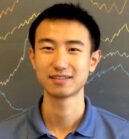# Jiaoyang Huang• Assistant Professor of Statistics and Data Science

## Contact Information

309 Academic Research Building
265 South 37th Street

Research Interests: Probability theory and its applications to problems from statistical physics, combinatorics, computer science and statistics.

## Education

Ph.D. in Mathematics, Harvard University, 2019

B.S. in Mathematics, Massachusetts Institute of Technology, 2014

B.S. in Computer Science and Technology, Tsinghua University, 2011

## Academic Positions Held

Simons Junior Fellow (Postdoc Associate), Courant Institute of Mathematical Sciences, New York University, 2020-2022

Member, Institute for Advanced Study, 2019-2020

## Current Courses (Fall 2022)

• ### MATH6480 - Probability Theory

Measure theoretic foundations, laws of large numbers, large deviations, distributional limit theorems, Poisson processes, random walks, stopping times.

MATH6480401

• ### STAT9300 - Probability Theory

Measure theoretic foundations, laws of large numbers, large deviations, distributional limit theorems, Poisson processes, random walks, stopping times.

STAT9300401 ( Syllabus )

• ### AMCS6481 - Probability Theory

Measure theoretic foundations, laws of large numbers, large deviations, distributional limit theorems, Poisson processes, random walks, stopping times.

AMCS6481401

## All Courses

• ### AMCS6481 - Probability Theory

Measure theoretic foundations, laws of large numbers, large deviations, distributional limit theorems, Poisson processes, random walks, stopping times.

• ### MATH6480 - Probability Theory

Measure theoretic foundations, laws of large numbers, large deviations, distributional limit theorems, Poisson processes, random walks, stopping times.

• ### STAT9300 - Probability Theory

Measure theoretic foundations, laws of large numbers, large deviations, distributional limit theorems, Poisson processes, random walks, stopping times.

## Activity

### Latest Research

Jiaoyang Huang (2022), Invertibility of Adjacency Matrices for Random d-Regular Graphs, Duke Mathematical Journal, 170 (18), pp. 3977-4032.
All Research